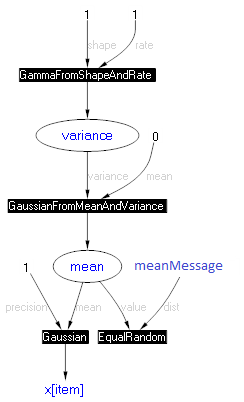Skip to main content

Infer.NET user guide

## Online learning

Bayesian inference makes it easy to update the parameters of a model as data arrives one at a time. In principle, you just use yesterday’s posterior as today’s prior. If the prior in your model has the same type as the posterior you are inferring, then this is straightforward. If the prior has a different type (for example it is specified using multiple factors and variables of its own), then you need a different approach. This document describes both methods.

As a concrete example, suppose you want to learn the mean of Gaussian as data arrives one at a time. If all of the data were available together, then you could provide it all to Infer.NET and this would give the best results. We will assume that your goal is to get results as close as possible to processing the data together. To do that, we need to mimic the operations that Infer.NET would have performed if it were performing one iteration over the data.

If the prior for the mean is a fixed Gaussian distribution, then you are in the simple case. We’ll assume that the online learning is performed over a set of data batches, and the parameter mean is shared across all of them. What you do after training on each data batch is to infer the posterior of mean and plug it in as the new prior for the next batch. Thus, you can think of meanPrior as the summary of what you’ve learnt “so far” (or “up to the current batch”).

When the prior is a more elaborate collection of factors and variables, you can no longer store your current knowledge in it in such a way. Suppose the variable mean now has a Laplacian prior, i.e. a Gaussian distribution whose mean is zero but whose variance is drawn from a Gamma. The simple approach does not work here since the posterior will be projected onto a Gaussian but your desired prior is not Gaussian. Since your goal is to get results as close as possible to processing the data together, you need to keep the form of the prior intact.

To process the batches sequentially, you need to add a new “accumulator” of your knowledge (an equivalent to what meanPrior was used for in the non-hierarchical case). We’ll call this meanMessage. It stores the product of all messages sent to mean by the previous batches. You attach this variable to mean by using the special factor ConstrainEqualRandom. This has the effect of multiplying meanMessage into the posterior of mean. The resulting factor graph is shown below.Initially, meanMessage should be Uniform, since there are no previous batches. After each batch, you need to store there the message sent upward to mean, which also happens to be the marginal of mean divided by its prior. Infer.NET has a special QueryType that gives you this ratio directly:

``````meanMessage.ObservedValue = engine.Infer<Gaussian>(mean, QueryTypes.MarginalDividedByPrior);
``````

However, you need to give the compiler a hint that you’ll be doing this:

``````mean.AddAttribute(QueryTypes.MarginalDividedByPrior);
``````

The complete code for online learning of a Gaussian with a Laplacian mean can be found in OnlineLearning.cs, along with other examples. The output of this code should be:

``````mean after batch 0 = Gaussian(0.9045, 0.6301)
mean after batch 1 = Gaussian(1.798, 0.4907)
mean after batch 2 = Gaussian(2.529, 0.3333)
mean after batch 3 = Gaussian(3.146, 0.25)
mean = Gaussian(3.146, 0.25) should be Gaussian(3.146, 0.25)
``````

The last line shows that, by using the accumulator, the posterior distribution obtained by processing the data one at a time matches the posterior distribution obtained by processing the data together.

This approach generalizes to any number of shared parameters. For each parameter, you store its upward messages in an accumulator, and attach the accumulator to the parameter via ConstrainEqualRandom. This approach is the closest you can get to processing the data together because it duplicates exactly what would happen internally if you ran EP for one iteration over the data.

The approach described here is a simplified version of what Shared Variables do automatically. You can use Shared Variables for online learning as well, but inference will be slightly less efficient since Shared Variables are designed for multiple passes through the data.